# numpy.meshgrid()理解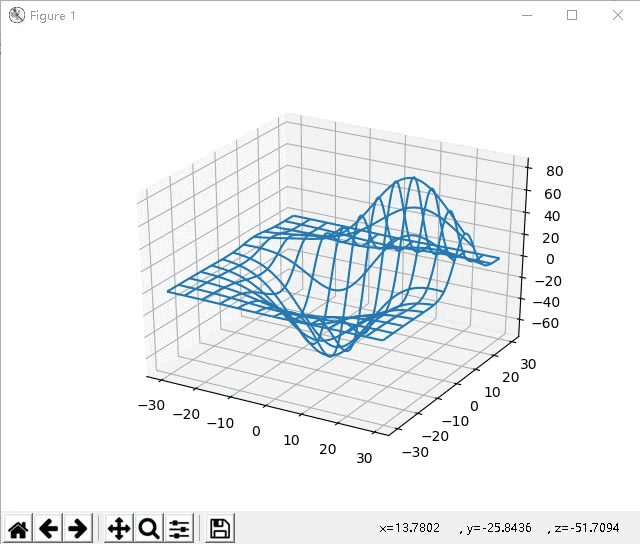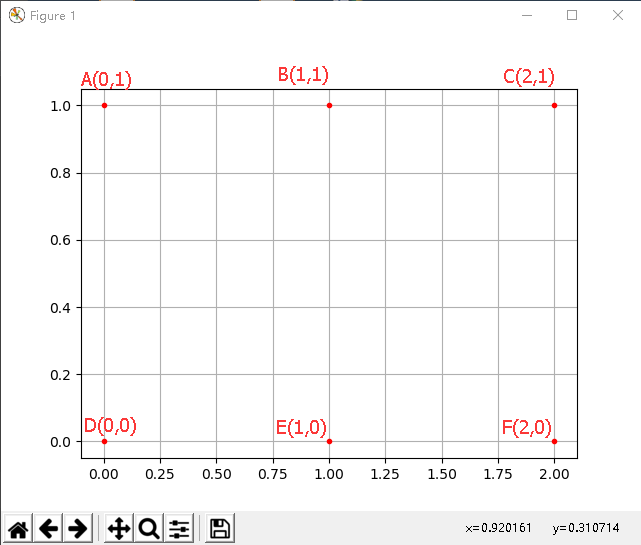A,B,C,D,E,F是6个网格点，坐标如图，如何用矩阵形式（坐标矩阵）来批量描述这些点的坐标呢？

X = [ 0 1 2 0 1 2 ] X= \left[ \begin{matrix} 0 &amp; 1 &amp; 2 \\ 0 &amp; 1 &amp; 2 \end{matrix} \right]
Y = [ 1 1 1 0 0 0 ] Y= \left[ \begin{matrix} 1 &amp; 1 &amp; 1 \\ 0 &amp; 0 &amp; 0 \end{matrix} \right]

import numpy as np
import matplotlib.pyplot as plt

x = np.array([[0, 1, 2], [0, 1, 2]])
y = np.array([[0, 0, 0], [1, 1, 1]])

plt.plot(x, y,
color='red',  # 全部点设置为红色
marker='.',  # 点的形状为圆点
linestyle='')  # 线型为空，也即点与点之间不用线连接
plt.grid(True)
plt.show()plt.plot(x, y,
marker='.',  # 点的形状为圆点
markersize=10,  # 点设置大一点，看着清楚
linestyle='-.')  # 线型为点划线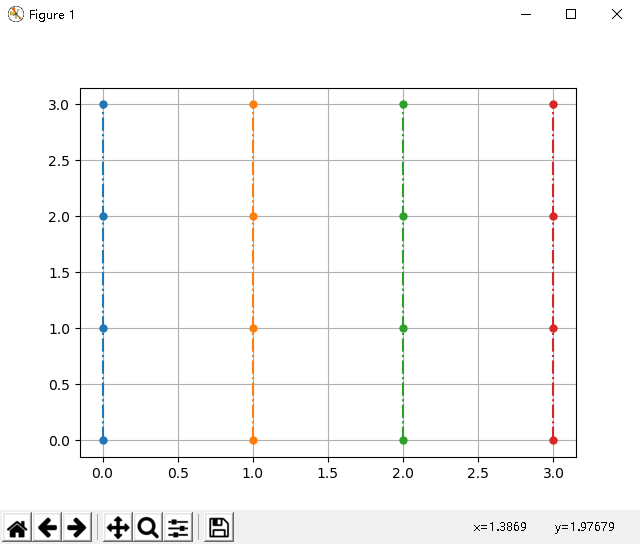import numpy as np
import matplotlib.pyplot as plt

x = np.array([[0, 1, 2, 3],
[0, 1, 2, 3],
[0, 1, 2, 3],
[0, 1, 2, 3]])
y = np.array([[0, 0, 0, 0],
[1, 1, 1, 1],
[2, 2, 2, 2],
[3, 3, 3, 3]])

plt.plot(x, y,
marker='.',  # 点的形状为圆点
markersize=10,  # 点设置大一点，看着清楚
linestyle='-.')  # 线型为点划线
plt.grid(True)
plt.show()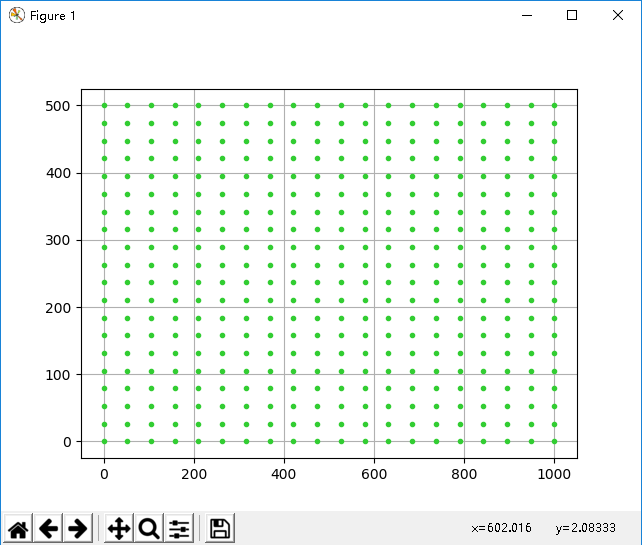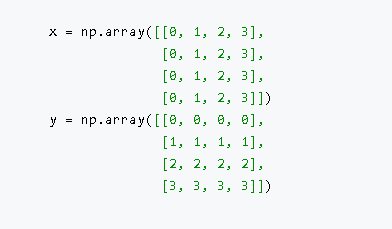import numpy as np
import matplotlib.pyplot as plt

x = np.array([0, 1, 2])
y = np.array([0, 1])

X, Y = np.meshgrid(x, y)
print(X)
print(Y)

plt.plot(X, Y,
color='red',  # 全部点设置为红色
marker='.',  # 点的形状为圆点
linestyle='')  # 线型为空，也即点与点之间不用线连接
plt.grid(True)
plt.show()

# 从输出的结果来看，两种方法生成的坐标矩阵一毛一样。
[[0 1 2]
[0 1 2]]
[[0 0 0]
[1 1 1]]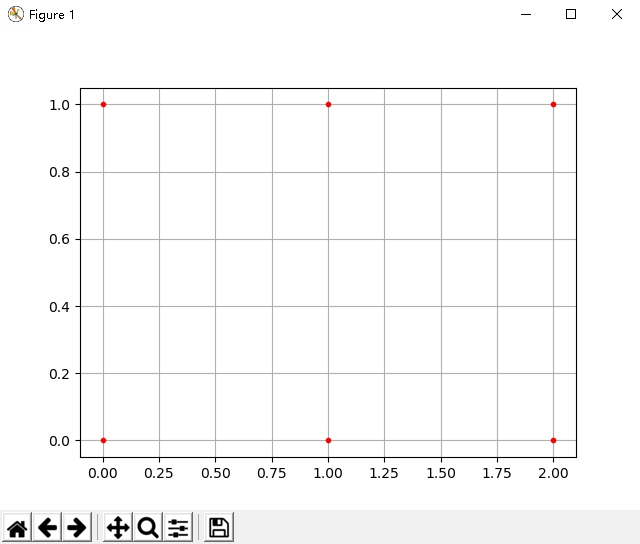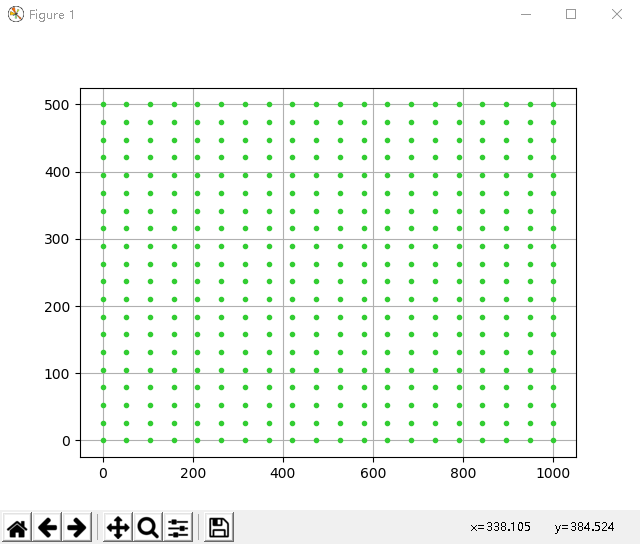import numpy as np
import matplotlib.pyplot as plt

x = np.linspace(0,1000,20)
y = np.linspace(0,500,20)

X,Y = np.meshgrid(x, y)

plt.plot(X, Y,
color='limegreen',  # 设置颜色为limegreen
marker='.',  # 设置点类型为圆点
linestyle='')  # 设置线型为空，也即没有线连接点
plt.grid(True)
plt.show()


https://www.cnblogs.com/black-mamba/p/9186965.html

S e l f - D i s c i p l i n e    a n d    S o c i a l    C o m m i t m e n t Self\text{-}Discipline \ \ and \ \ Social \ \ Commitment

08-09179
01-05207
01-177614
10-304万+
08-173万+
03-093503
07-0599
09-01158
03-28460
02-19249
05-141551
11-01293
03-088614
12-061687
03-10114
05-061022
01-1722万+

### “相关推荐”对你有帮助么？

•非常没帮助
•没帮助
•一般
•有帮助
•非常有帮助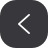被折叠的  条评论 为什么被折叠?到【灌水乐园】发言¥2 ¥4 ¥6 ¥10 ¥20余额支付 (余额：-- )扫码支付获取中扫码支付点击重新获取扫码支付1.余额是钱包充值的虚拟货币，按照1:1的比例进行支付金额的抵扣。
2.余额无法直接购买下载，可以购买VIP、C币套餐、付费专栏及课程。余额充值